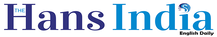The pressure & gas lawsx
Highlights

Everything that has mass, from an elephant to a sugar molecule, is considered to be matter if it can exist as a solid, liquid, or gas.

Everything that has mass, from an elephant to a sugar molecule, is considered to be matter if it can exist as a solid, liquid, or gas.

Gases are formed by small molecules in constant rapid, random motion; they have a much lower density (concentration of molecules) than either solids or liquids.

You can observe all three states of matter in water: from a liquid, water (H2O) will turn into solid ice when it freezes or will evaporate as gas when it's heated to boiling.

One of the important properties of gas is that it expands when it gets hot and contracts when it cools.

This means that when a gas is heated, the individual molecules that form it will move faster and spread further apart from each other.

The gas also gets lighter as it gets hotter, since the molecules are less densely concentrated.

The expanding property of gas is what enables hot air balloons to inflate and fly.

Hot air going into the balloon has a less dense concentration of molecules, making the inflated balloon lighter than the cooler, denser air around it.

That denser outer air buoys up the balloon and allows it to float.

Conversely, the affect of cooling air will bring the balloon back down—the air molecules inside the balloon move closer together when cooled, making the air in the balloon no longer lighter than the surrounding air.

That's why a cold, damp cloud or fog can be dangerous for someone in a hot air balloon.

(Although it might seem like air is weightless, it's actually formed by gases and has mass like any other matter.

Usually the air is made up of 78% nitrogen, 21% oxygen, and 1% carbon dioxide and noble gases.

The density of air is 0.075 pounds per cubit foot at 70 °F and one atmosphere of pressure.)

The pressure & gas laws: Pressure (P) can be described mathematically as "force (F) divided by the area over which that force is applied," or P=F/A.

Pressure is measured in pascals (Pa), named in honor of the 17th-century scientist and philosopher Blaise Pascal, who showed that confined liquids transmit pressure equally in all directions.

Pressure can also be measured in pounds per square inch.

You can use this formula to find the pressure exerted on your feet by your body; divide your weight in pounds by the number of square inches that your feet cover.

If you weigh 100 pounds and the surface area of your feet is approximately 50 inches, then the pressure is around two lbs/in2.

(To find total surface area of your foot, multiply average length by average width for each foot and then add those together.)

The larger the area, the less pressure per square inch. There are advantages sometimes to having big feet!

There are several scientific laws to describe the behavior of gases.

Boyle's Law says that under constant temperature, the product of pressure (P) times volume (V) must remain constant, or PV=constant.

This means that if one increases, the other must decrease (if P↑ then V↓).

Charles' Law says that under constant pressure, the quotient of volume (V) divided by temperature (T) is constant, or V/T=constant.Next Story
More Stories